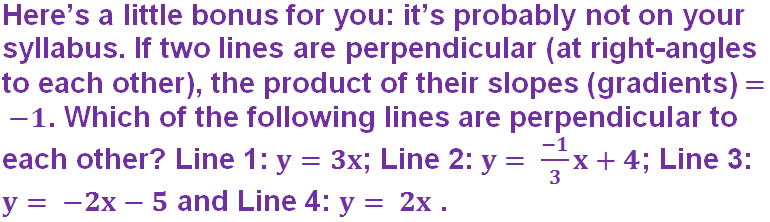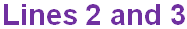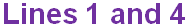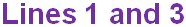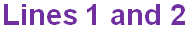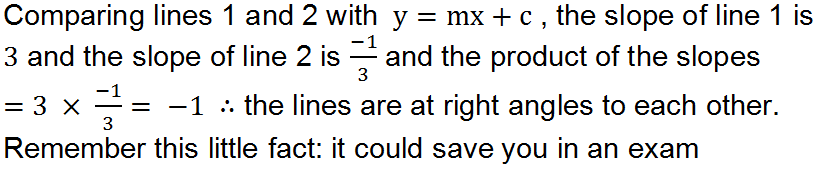If you need to improve your maths skills, try this quiz on straight lines.

# Straight Line (H)

Lots of real life situations can be modelled as a straight line, and if you are studying GCSE subjects as diverse as Physics, Business Studies, Geography or Engineering you are more than likely to come across it in one form or another! Support your success in other subjects by acing this GCSE Maths quiz!

You should be familiar with the format for the general equation of the straight line (y = mx + c) and the information you can obtain from it (gradient and y-intercept). If you are a bit unsure, try the Straight Line (F) quiz first. What can you do with that information? If you are told a second line is parallel to it, this implies they have the same gradient. If the second line has the same y-intercept, then the c value is the same.

But what if the information you are given isn’t so straightforward? What if you know the gradient and the coordinates of a point it passes through, but this point isn’t on the y-axis? A good understanding of the link between the algebra and the geometry will help you solve these types of problems.

Let the coordinates of the point it passes through be (x1, y1). Substitute these, along with the gradient, into the general equation y - y1 = m(x - x1). Multiply out the brackets and tidy up.

You may be given even less information, such as only being told two points it passes through. In this case you firstly need to calculate the gradient (change in y over change in x), then use the previous technique with one of the points.

Equations may be given in the format ax + by = c. This is useful if you want to find both the x- and y-intercepts, but you will need to rearrange it to identify the gradient.

Question 1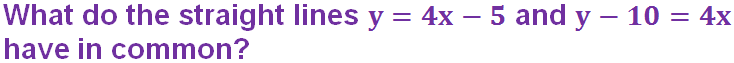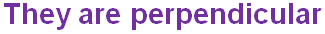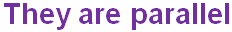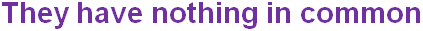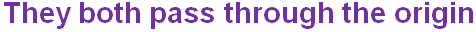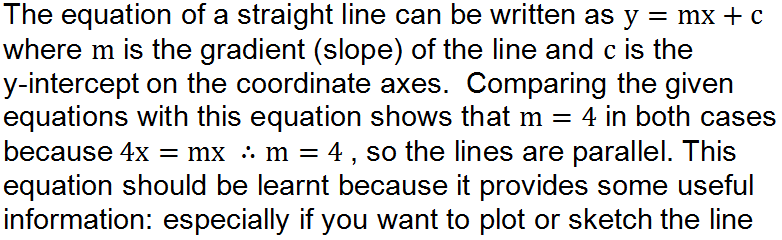Question 2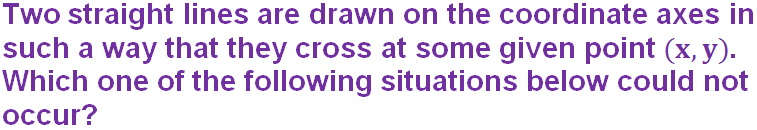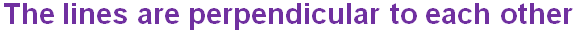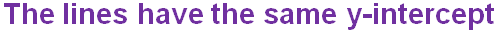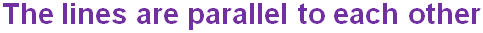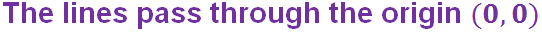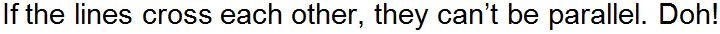Question 3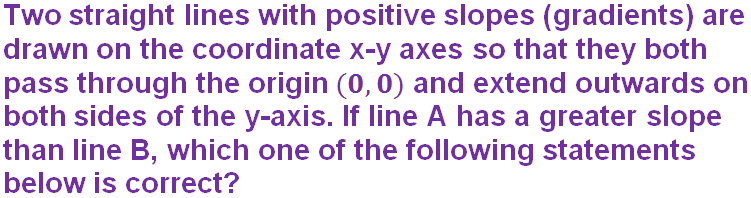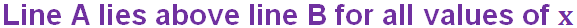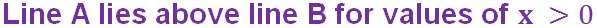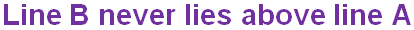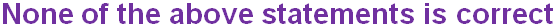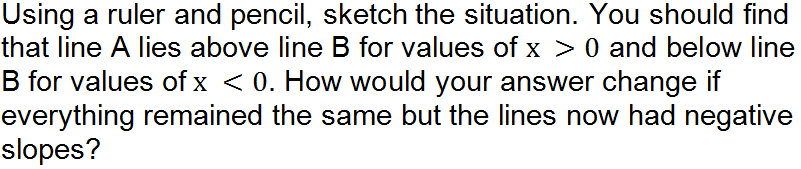Question 4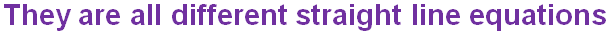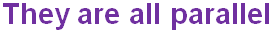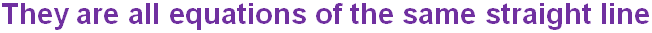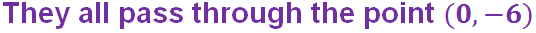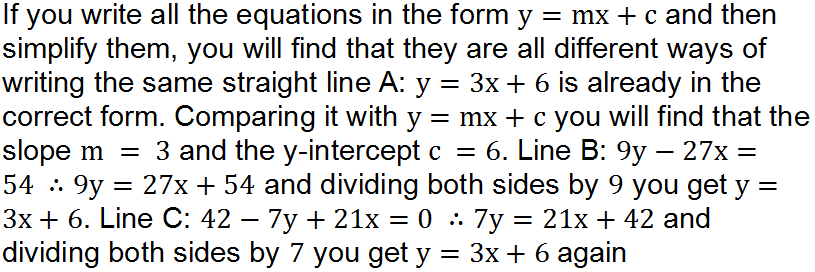Question 5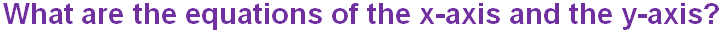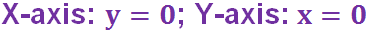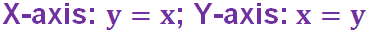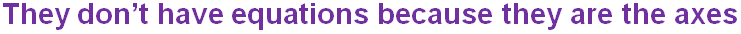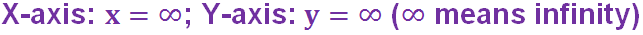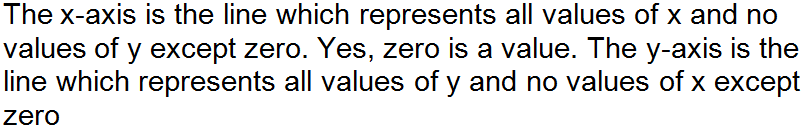Question 6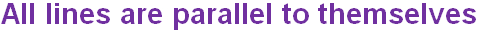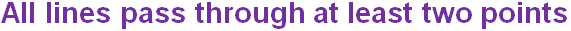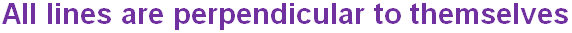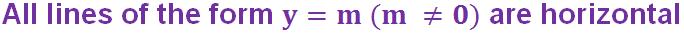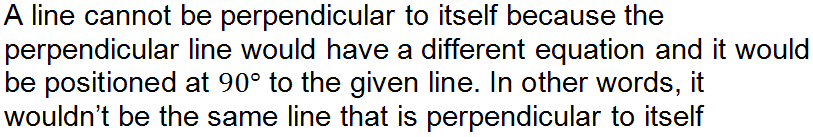Question 7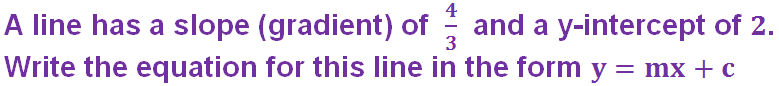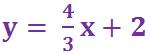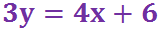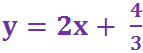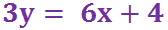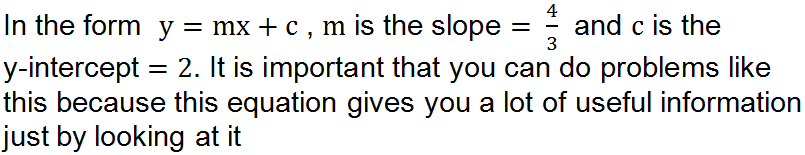Question 8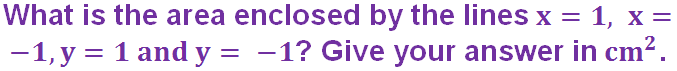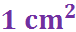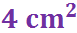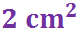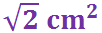Question 9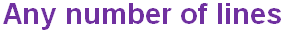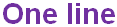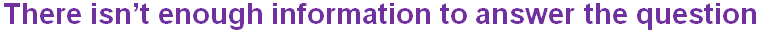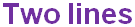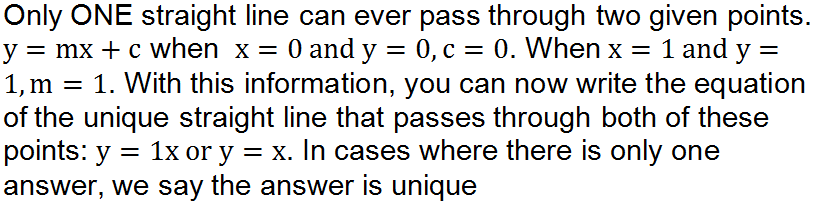Question 10